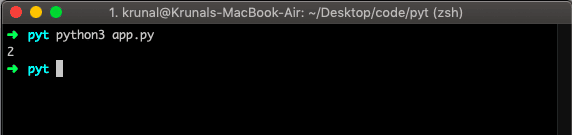# Python list index: The Complete Guide

If any element not presented is searched, it returns the ValueError. The index() method returns the lowest index in the list that element appears.

## Python List Index

Python list index() is a built-in function that searches an element in the list and returns its index. The list index() method returns the index of the element in the list.

### Syntax

The syntax for the index() method is the following.

`list.index(element)`

### Parameters

The element parameter is required and is the item searched for in the list.

Let us take an example.

```# app.py

GoT = ['Daenerys', 'Jon', 'Tyrion']
elementIndex = GoT.index('Jon')
print(elementIndex)```

So, the index() method returns an index, and in the above example, it returns Jon’s index, which is 1.## Find Index of Item Which Doesn’t Exist

If we find an index of an element not present in the list, it will return a ValueError. Let us see the following code.

```# app.py

GoT = ['Daenerys', 'Jon', 'Tyrion']
elementIndex = GoT.index('Arya')
print(elementIndex)```

See the output.## Find an index of tuple and list inside a list

Let us find an index of a tuple in the list. Let us look at the following example.

```# app.py

GoT2 = ['Daenerys', 'Jon', ('Briane', 'Tormund') , 'Tyrion']
elementIndex2 = GoT2.index(('Briane', 'Tormund'))
print(elementIndex2)```

See the output.So, it works the same as a single element in the list because it behaves as a single element in a list.

That’s it for this tutorial.

This site uses Akismet to reduce spam. Learn how your comment data is processed.